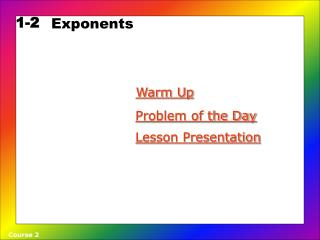DownloadDownload Presentation1-2

# 1-2

Download Presentation## 1-2

- - - - - - - - - - - - - - - - - - - - - - - - - - - E N D - - - - - - - - - - - - - - - - - - - - - - - - - - -
##### Presentation Transcript

1. 1-2 Exponents Warm Up Problem of the Day Lesson Presentation Course 2

2. Warm Up Simplify. 1.2 · 2 · 2 2. 3 · 3 · 3 · 3 3. 5 · 5 · 5 4. 4 · 4 · 4 5. 6 · 6 · 6 · 6 · 6 8 81 125 64 7,776

3. Problem of the Day You intend to place water lilies in the pond in your backyard. A water lily doubles in size every day. From the time you install the first lily until the entire surface of the pond is covered will take 20 days. How long will it take for the pond to be half covered? 19 days

4. Learn to represent numbers by using exponents. Vocabulary power exponent base factor

5. A DNA molecule makes a copy of itself by splitting in half. Each half becomes a molecule that is identical to the original. The molecules continue to split so that the two become four, the four become eight, and so on. 2 · 2 · 2 = 8 Each time DNA copies itself, the number of molecules doubles. How many molecules are there after 4 copies? 2 · 2 · 2 · 2 = 16

6. 4 2 Base Exponent 2 · 2 · 2 · 2 = 16 This multiplication can also be written as a power, using a base and an exponent. The exponent tells how many times to use the baseas a factor. Reading Math Read 24 as “the fourth power of 2” or “2 to the fourth power.”

7. Find each value. A. 44 Use 4 as a factor 4 times. 44 = 4 · 4 · 4 · 4 = 256 B. 73 Use 7 as a factor 3 times. 73 = 7 · 7 · 7 = 343 C. 191 191 = 19 Use 19 as a factor 1 time.

8. Find each value. A. 33 B. 62 B. 141

9. Any number to the zero power, except zero is equal to 1. 60 = 1 100 = 1 190 = 1 Zero to the zero power is undefined, meaning that it does not exist.

10. To express a whole number as a power, write the number as a product of equalfactors. Then write the product using the base and an exponent. For example, 10,000 = 10 · 10 · 10 · 10 = 104.

11. Write each number using an exponent and the given base. A. 625, base 5 5 is used as a factor 4 times. 625 = 5 · 5 · 5 · 5 = 54 B. 64, base 2 2 is used as a factor 6 times. 64 = 2 · 2 · 2 · 2 · 2 · 2 = 26

12. Write each number as an exponent and the given base. A. 343, base 7 B. 243, base 3

13. On Monday, Erik tells 3 people a secret. The next day each of them tells 3 more people. If this pattern continues, how many people besides Erik will know the secret on Friday? 3 On Monday, 3 people know the secret. On Tuesday, 3 times as many people know as those who knew on Monday. On Wednesday, 3 times as many people know as those who knew on Tuesday. On Thursday, 3 times as many people know as those who knew on Wednesday. On Friday, 3 times as many people know as those who knew on Thursday. 3 · 3 3 · 3 · 3 3 · 3 · 3· 3 3 · 3 · 3 · 3 · 3

14. Each day the number of people is 3 times greater. 3 · 3 · 3 · 3 · 3 = 35 = 243 Monday Tuesday Wednesday Thursday Friday On Friday, 243 people besides Erik will know the secret.

15. In a game, a contestant had a starting score of one point. She doubled her score every turn for four turns. Write her score after four turns as a power. Then find her score. 2 After the first turn, she had points. After the second turn, she would have points. After the third turn, she would have points. After each turn, her point total is times greater. 2 · 2 · 2 · 2 = 24 = 16 points 4 8 2

16. Do you know… • In 52, what is the base? • What does the base tell you? • In 52, what is the exponent? • What does the exponent tell you? • When you find the value of 52, how do you know how many times to use the base as a factor? • Explain why any number to the first power equals that number. • Tell which power of 8 is equal to 26. Explain.

17. Lesson Quiz Find each value. 1. 73 3. 34 Write each number using an exponent and given base. 5. 125, base 5 6. 16, base 2 7. Find the volume of a cube if each side is 12 inches long. 343 2. 63 216 81 4. 85 32,768 53 24 1,728 in3